# Knowledge Base

## Solution 35314: Finding Critical Points Using the TI-Nspire™ CAS Family.

### How can critical points be found using the TI-Nspire CAS family?

The critical points of a function are the points where the derivative is equal to zero, reach a local minimum, or reach a local maximum. The following illustrates how to use the differentiate and solve functions to find critical points.

Example: Find the critical points using the solver and differentiation commands on the home screen for the following function:

f(x)= x^3+x^2-5x-5

The first step to finding the critical points is to differentiate the above function:

1) Press [home] and choose to add a Calculator.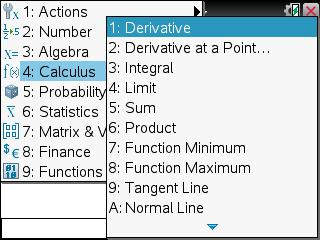3) Press [X] [tab].
4) Press [X] [^]  [tab].
5) Press [+] [X] [x2] [-]  [X] [-]  [,] [X] [)].
6) Press [enter].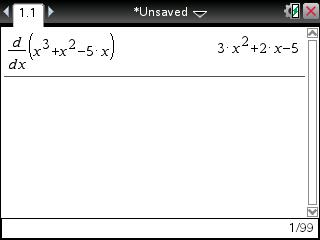To find one of the critical points the user will need to set the derivative (shown above) equal to zero and solve for x: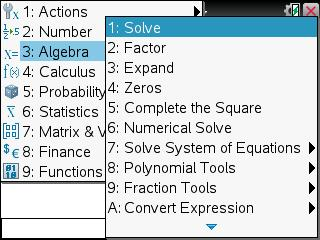2) Press  [X] [x2] [+]  [X] [-] .
3) Press [=]  [,] [X] [)].
4) Press [enter].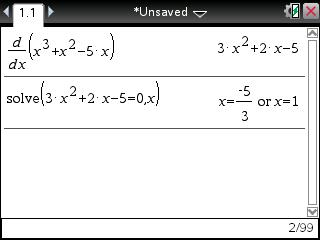Since there are two answers for the following function, there will be two sets of critical points on this function.

Now to solve for the additional points, plug x into the original equation:

1) Press [X] [^]  [tab].
2) Press [+] [X] [x2].
3) Press [-]  [X] [-] .
4) Press [ctrl] [=] and choose "|".
5) Press [X] [=] .
6) Press [enter].
7) Press [X] [^]  [tab].
8) Press [+] [X] [x2].
9) Press [-]  [X] [-] .
10) Press [ctrl] [=] and choose "|".
11) Press [X] [=] [ctrl] [÷].
12) Press [(-)]  [tab] .
13) Press [enter].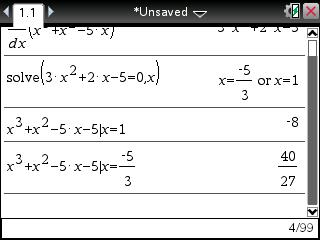The answers show the y coordinates for the two critical points on this function. So the critical values for the function f(x)= x^3+x^2-5x-5 are (-5/3,40/27) and (1,-8).

Please see the TI-Nspire CX CAS and TI-Nspire CAS guidebooks for additional information.